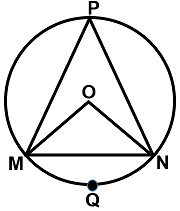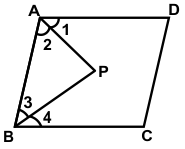﻿ Maths Olympiad (CMO) Sample Paper & Questions for Class 9

# CREST Mathematics Olympiad Class 9 Sample Paper

Sample PDF of CREST Mathematics Olympiad for Class 9:

## Syllabus:

Section 1: Number Systems, Polynomials, Coordinate Geometry, Linear Equations in Two Variables, Introduction to Euclid’s Geometry, Lines and Angles, Triangles, Quadrilaterals, Areas of Parallelograms and Triangles, Circles, Constructions, Heron’s Formula, Surface Areas and Volumes, Statistics, Probability.

Achievers Section: Higher Order Thinking Questions - Syllabus as per Section 1

 Q.1 Two years ago, the ratio of A's age to B's age at that time was 5:9. A's age three years ago was 13 years less than B's age six years ago. What is B's present age?
 Q.2 In measuring the sides of a rectangle, there is an excess of 5% on one side and 2% deficit on the other. Then the error per cent in the area is:
 Q.3 The numerator of a fraction is 4 less than its denominator. If the numerator is decreased by 2 and the denominator is increased by 1, then the denominator becomes eight times the numerator, then find the fraction.
 Q.4 An urn contains 6 blue and 'P' green balls. If the probability of drawing a green ball is double that of drawing a blue ball, then 'P' is equal to:
 Q.5 The ratio of the number of students in two classrooms, C1 and C2, is 2:3. It is observed that after shifting ten students from C1 to C2, the ratio is 3:7. Further, how many students have to be shifted from C2 to C1 for the new ratio to become 9:11?
 Q.6 The solution set formed by the regions x + y > 7 and x + y < 10 in the first quadrant represents a _________.
 Q.7 On simplifying (a + b)3 + (a - b)3 + 6a(a2 - b2) we get:
 Q.8 In the following figure , O is the centre of the circle. If ∠MPN = 55⁰, then find the value of: ∠MON + ∠OMN + 1/2 ∠MNOQ.9 In the triangle ABC, AB = 2 cm, BC = 3 cm and AC = 4 cm. D is the middle-point of AC. If a square is constructed with BD as one of its sides, what is the area of the square?
 Q.10 In the given figure, AP and BP are angle bisectors of ∠A and ∠B, respectively which meets at P on the parallelogram ABCD. Then 2∠APB =  ?